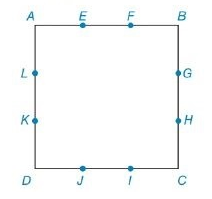Chapter 8.2, Problem 41EElementary Geometry For College St...

7th Edition
Alexander + 2 others
ISBN: 9781337614085

Solutions

Chapter
SectionElementary Geometry For College St...

7th Edition
Alexander + 2 others
ISBN: 9781337614085
Textbook Problem

For Exercises 41 and 42, the sides of square ABCD are trisected at the indicated points.Find the ratio: a) P E G I K P A B C D b) A E G I K A A B C DExercises 41 and 42

To determine

(a)

To find:

Ratio of the perimeter of the square EGIK to the square ABCD.

Explanation

Formula Used:

1. Pythagorean theorem for the right angle triangle ABC for the hypotenuse AC,

AC2=AB2+BC2.

2. The perimeter of a polygon is the sum of the lengths of all sides of the polygon. P=4s, where s is side length of the square.

Calculation:

It is given that the sides of square ABCD are trisected as shown in the below diagram.

Let the side length of the square be 3x units. Then the each part will measure x units.

Here EGIK is also a square, because the inner square EGIK intersects the outer square at the same first trisected point in all the sides of the outer square ABCD.

As EGIK is a square, each side of ABCD can also be split into two parts. That is, x and 2x respectively. Therefore, AE=BG=IC=DK=x and EB=GC=ID=KA=2x

To determine

(b)

To find:

Ratio of the area of the square EGIK to the square ABCD.

Still sussing out bartleby?

Check out a sample textbook solution.

See a sample solution

The Solution to Your Study Problems

Bartleby provides explanations to thousands of textbook problems written by our experts, many with advanced degrees!

Get Started

What are the two requirements for a random sample?

Essentials of Statistics for The Behavioral Sciences (MindTap Course List)

In Exercises 4562, find the values of x that satisfy the inequality (inequalities). 61. x2x12

Applied Calculus for the Managerial, Life, and Social Sciences: A Brief Approach

Rewritten as an iterated integral in polar coordinates,

Study Guide for Stewart's Multivariable Calculus, 8th

For , f′(x) =

Study Guide for Stewart's Single Variable Calculus: Early Transcendentals, 8th